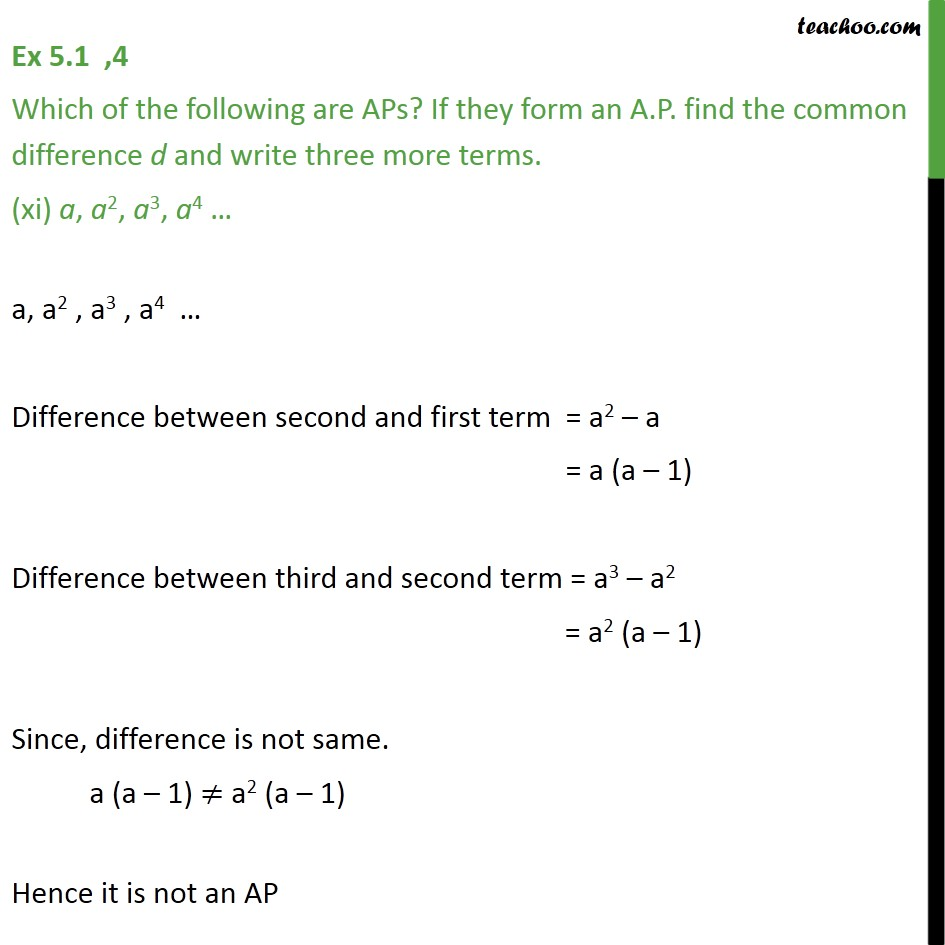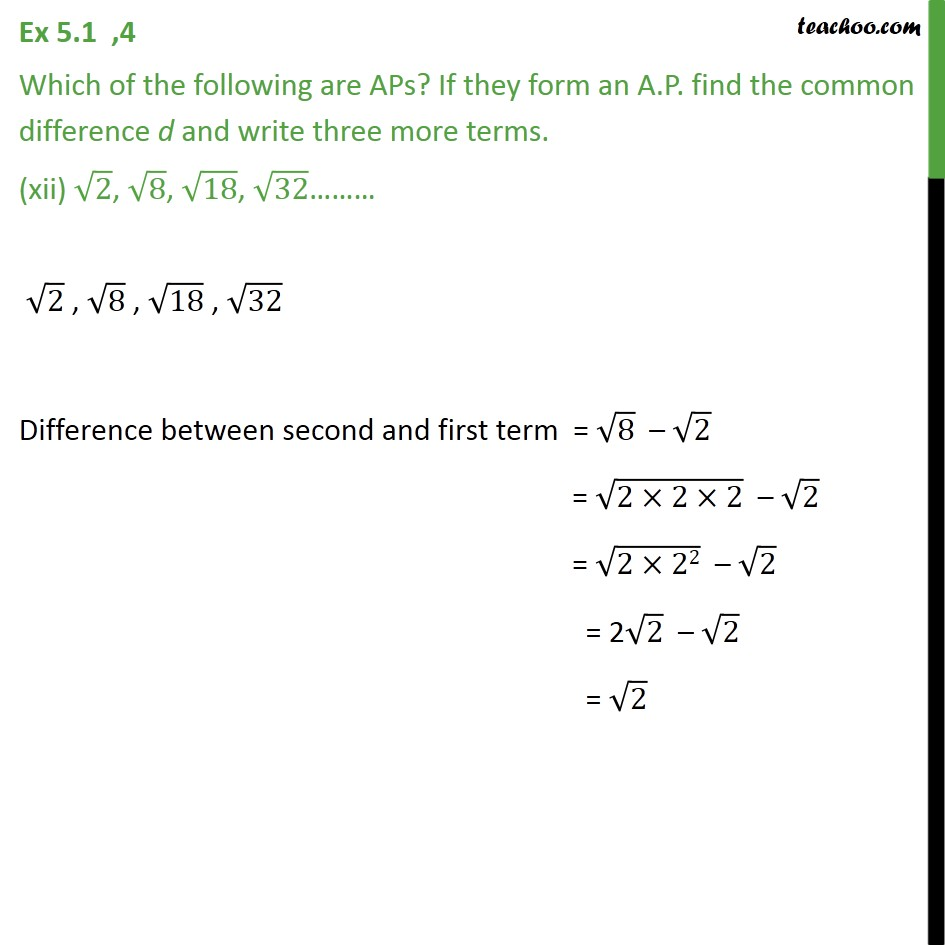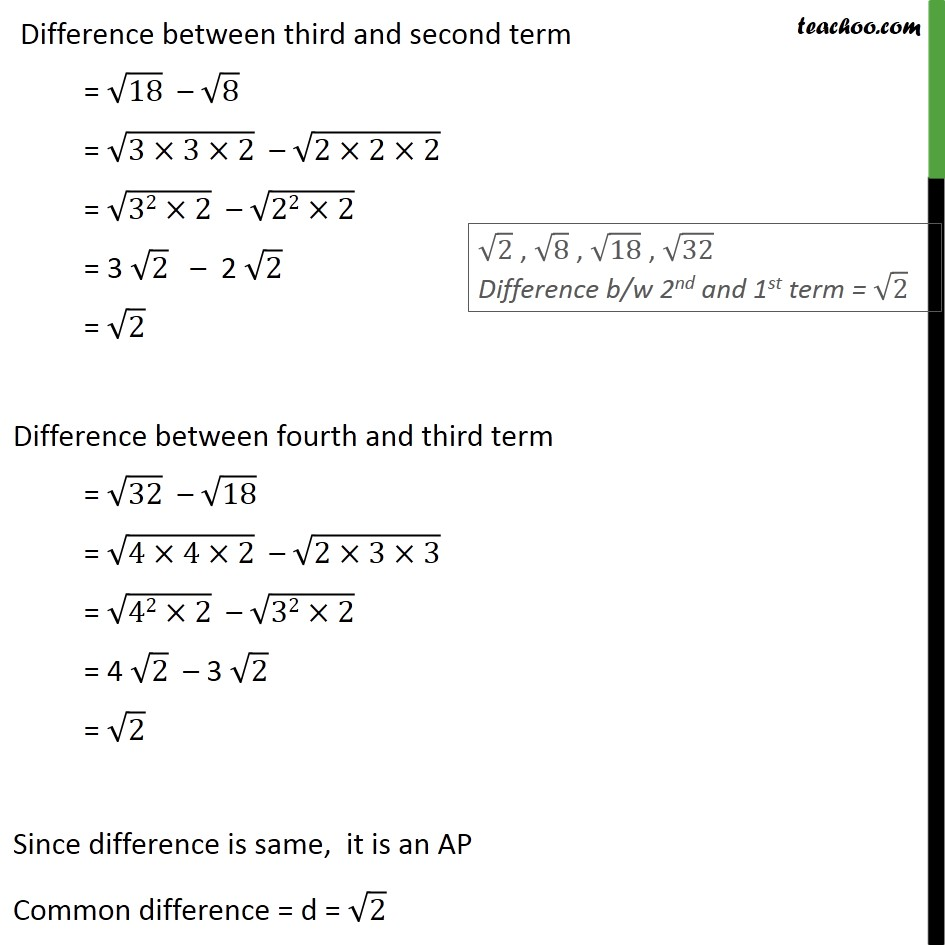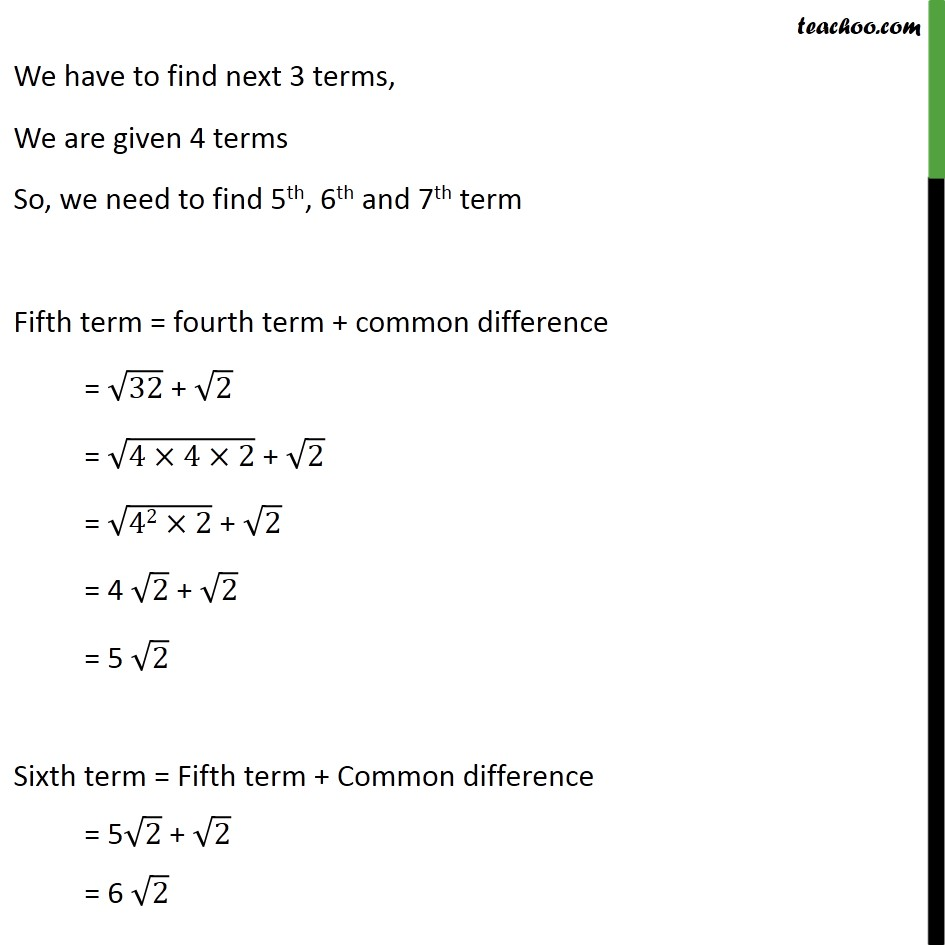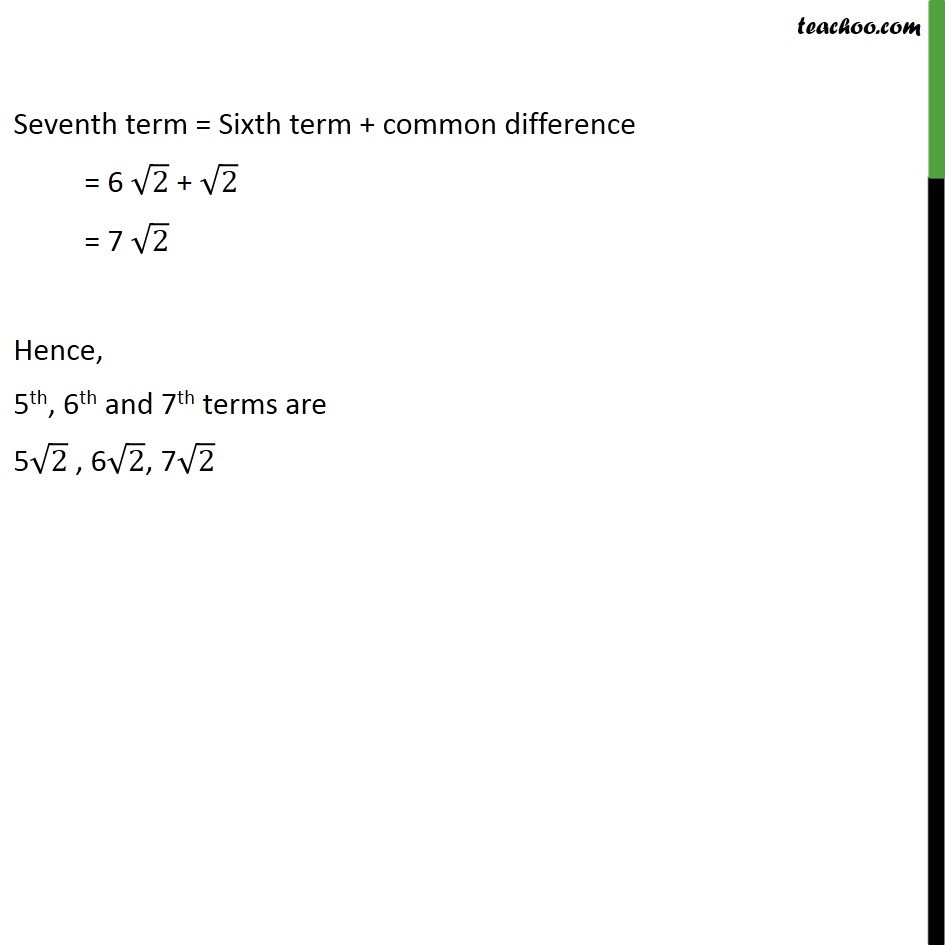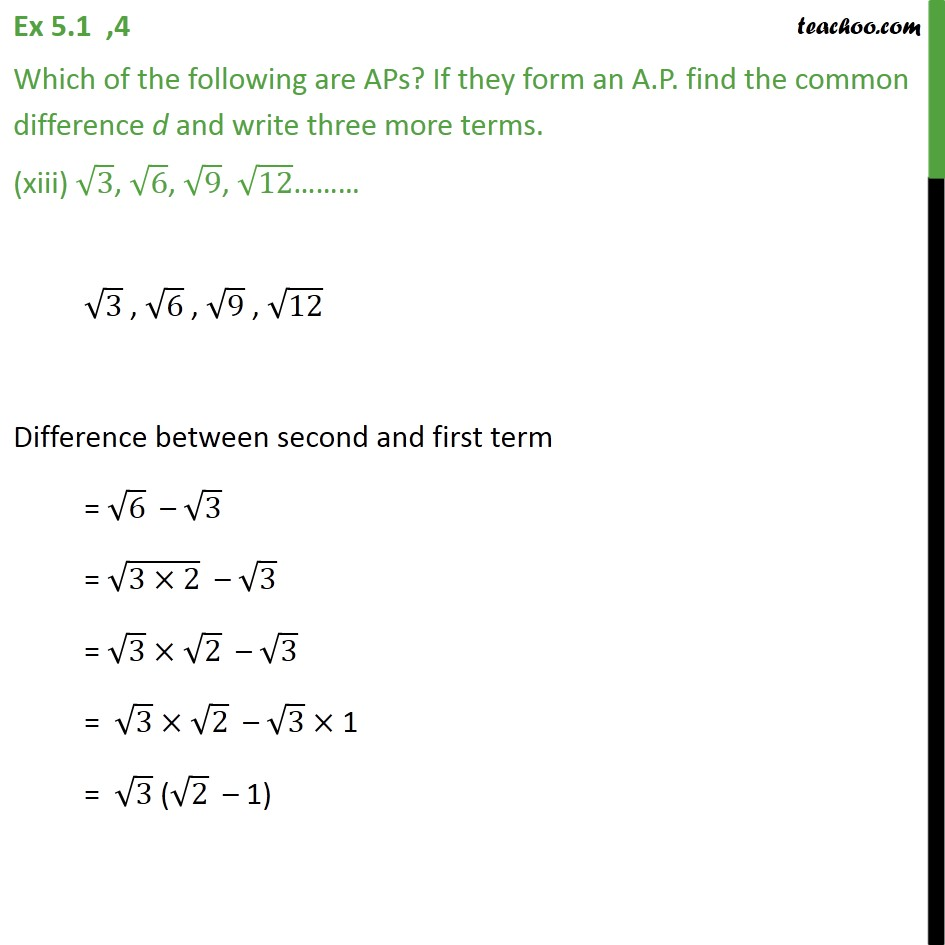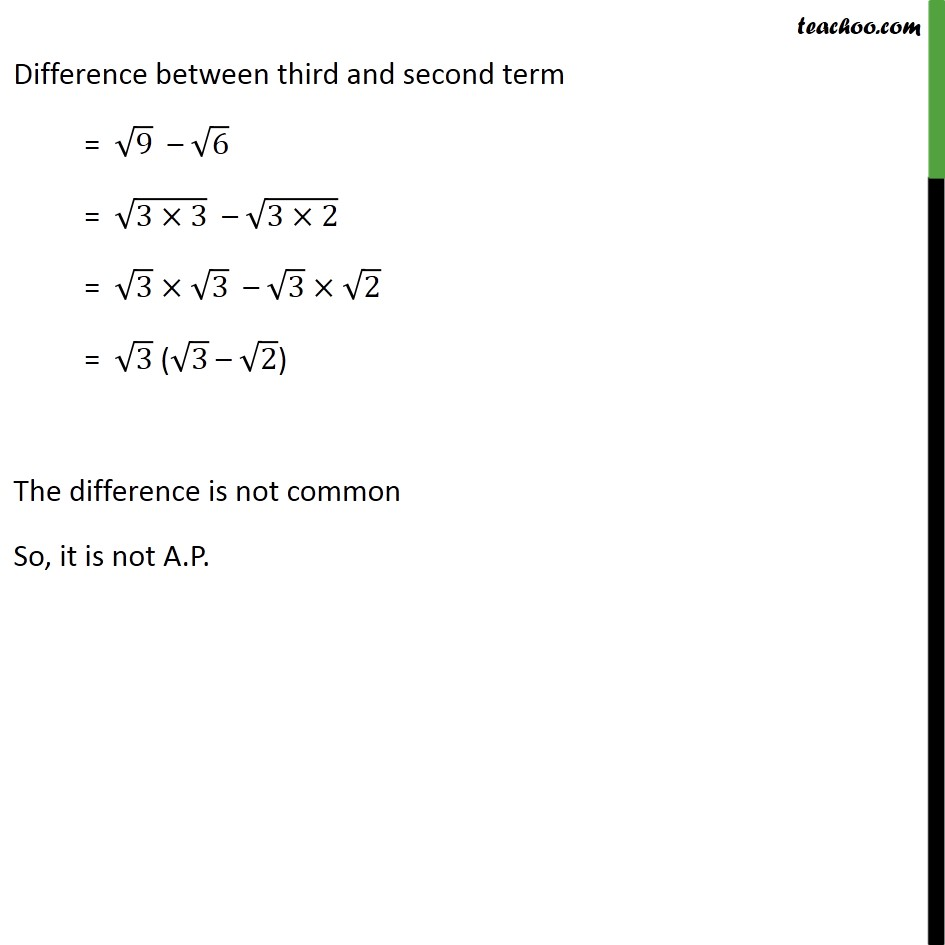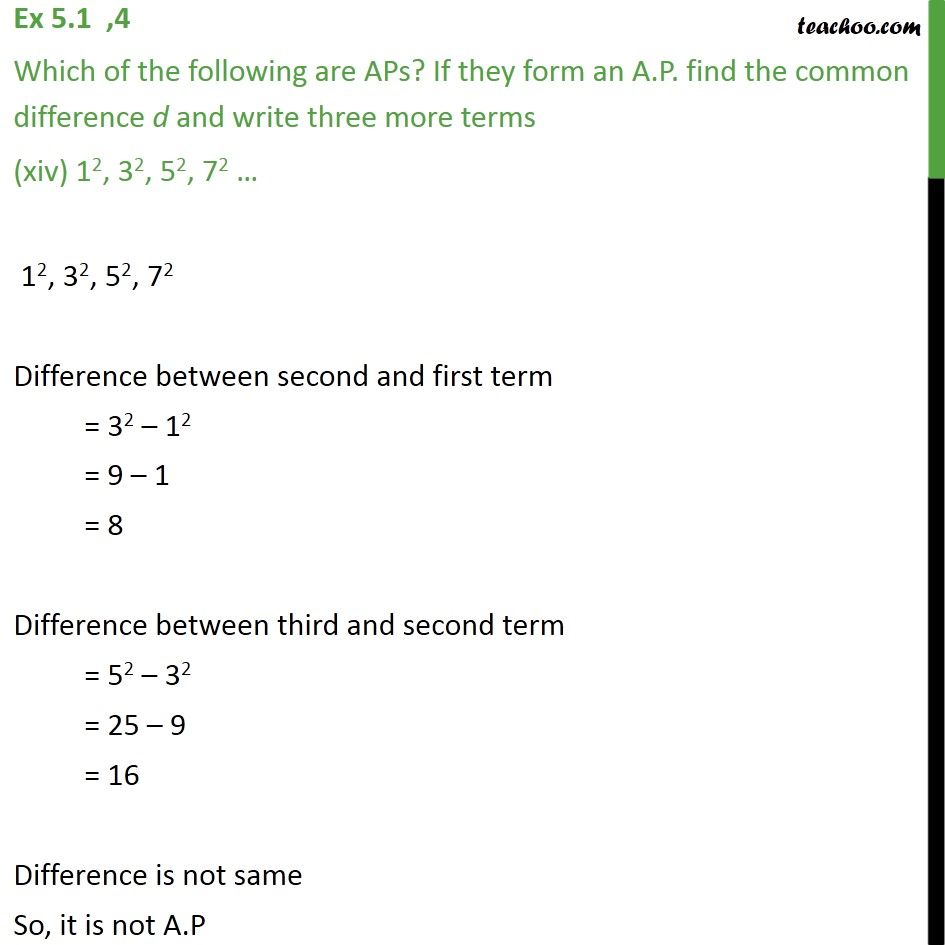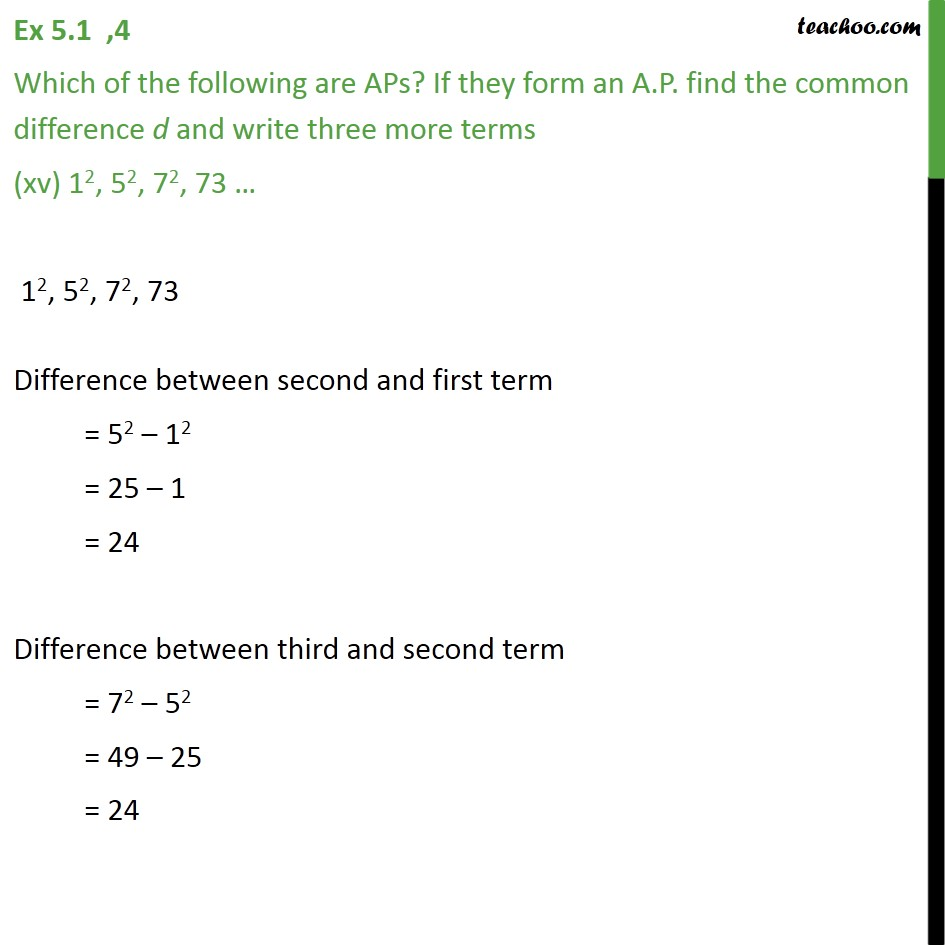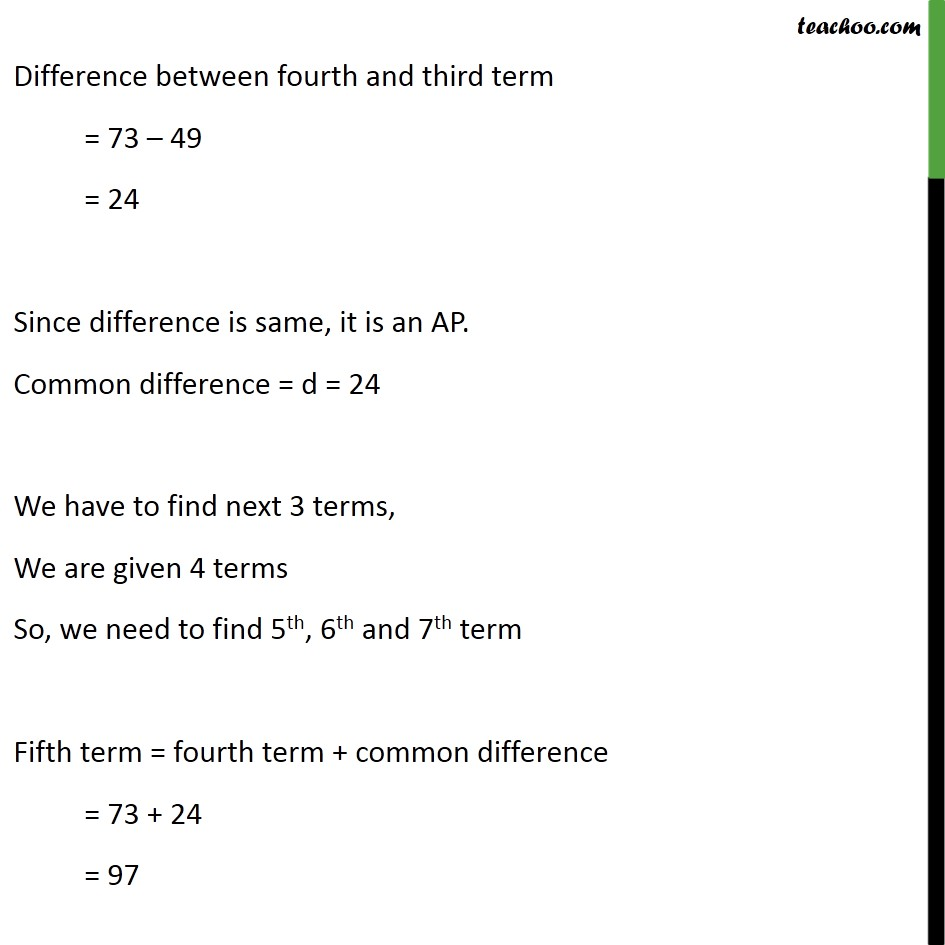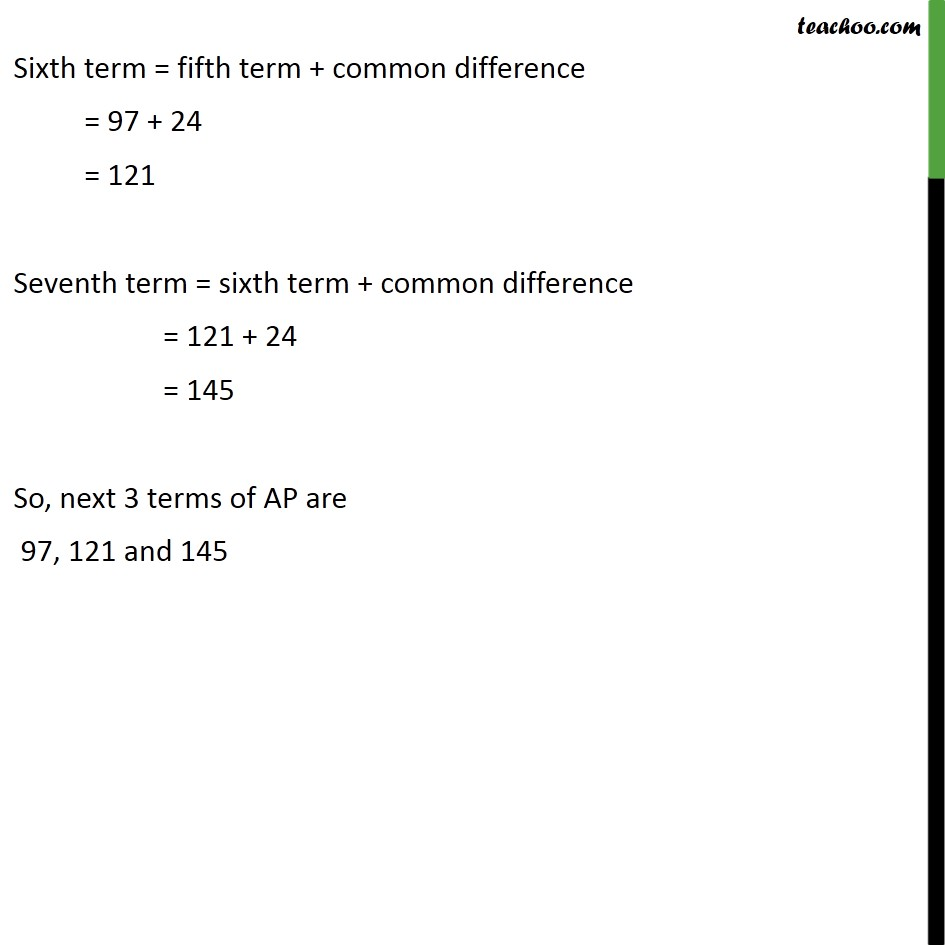1. Chapter 5 Class 10 Arithmetic Progressions
2. Concept wise
3. Checking if AP or not and finding a, d

Transcript

Ex 5.1 ,4 Which of the following are APs? If they form an A.P. find the common difference d and write three more terms. (xi) a, a2, a3, a4 a, a2 , a3 , a4 Difference between second and first term = a2 a = a (a 1) Difference between third and second term = a3 a2 = a2 (a 1) Since, difference is not same. a (a 1) a2 (a 1) Hence it is not an AP Ex 5.1 ,4 Which of the following are APs? If they form an A.P. find the common difference d and write three more terms. (xii) 2, 8, 18, 32 2 , 8 , 18 , 32 Difference between second and first term = 8 2 = (2 2 2) 2 = (2 22) 2 = 2 2 2 = 2 Difference between third and second term = 18 8 = (3 3 2) (2 2 2) = (32 2) (22 2) = 3 2 2 2 = 2 Difference between fourth and third term = 32 18 = (4 4 2) (2 3 3) = (42 2) (32 2) = 4 2 3 2 = 2 Since difference is same, it is an AP Common difference = d = 2 We have to find next 3 terms, We are given 4 terms So, we need to find 5th, 6th and 7th term Fifth term = fourth term + common difference = 32 + 2 = (4 4 2) + 2 = (42 2) + 2 = 4 2 + 2 = 5 2 Sixth term = Fifth term + Common difference = 5 2 + 2 = 6 2 Seventh term = Sixth term + common difference = 6 2 + 2 = 7 2 Hence, 5th, 6th and 7th terms are 5 2 , 6 2, 7 2 Ex 5.1 ,4 Which of the following are APs? If they form an A.P. find the common difference d and write three more terms. (xiii) 3, 6, 9, 12 3 , 6 , 9 , 12 Difference between second and first term = 6 3 = (3 2) 3 = 3 2 3 = 3 2 3 1 = 3 ( 2 1) Difference between third and second term = 9 6 = (3 3) (3 2) = 3 3 3 2 = 3 ( 3 2) The difference is not common So, it is not A.P. Ex 5.1 ,4 Which of the following are APs? If they form an A.P. find the common difference d and write three more terms (xiv) 12, 32, 52, 72 12, 32, 52, 72 Difference between second and first term = 32 12 = 9 1 = 8 Difference between third and second term = 52 32 = 25 9 = 16 Difference is not same So, it is not A.P Ex 5.1 ,4 Which of the following are APs? If they form an A.P. find the common difference d and write three more terms (xv) 12, 52, 72, 73 12, 52, 72, 73 Difference between second and first term = 52 12 = 25 1 = 24 Difference between third and second term = 72 52 = 49 25 = 24 Difference between fourth and third term = 73 49 = 24 Since difference is same, it is an AP. Common difference = d = 24 We have to find next 3 terms, We are given 4 terms So, we need to find 5th, 6th and 7th term Fifth term = fourth term + common difference = 73 + 24 = 97 Sixth term = fifth term + common difference = 97 + 24 = 121 Seventh term = sixth term + common difference = 121 + 24 = 145 So, next 3 terms of AP are 97, 121 and 145

Checking if AP or not and finding a, d